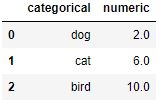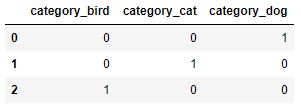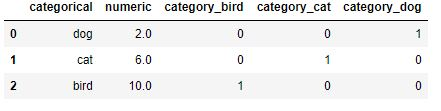## Python One Hot Encoding with Pandas Made Simple

### Introduction

If you have been using machine learning, you will sooner rather than later realize that machine learning algorithms require numerical inputs. Unlucky for us, our features will come in various forms. Some will be continuous, others categorical in numeric or text format. Machine learning algorithms cannot work with variables in text form, we must perform certain preprocessing steps to get our data in the right format.

How do we deal with these categorical variables? Worry no more! In this blog post I will explain how to deal with these categorical variables by using a technique known as one hot encoding.

After reading this blog post you should be able to:

• Know what is One Hot Encoding

• Perform One Hot Encoding with Pandas### One Hot Encoding Overview

One hot encoding is the technique to convert categorical values into a 1-dimensional numerical vector. The resulting vector will have only one element equal to 1 and the rest will be 0. The 1 is called Hot and the 0’s are Cold. This is where its name of one hot encoding comes from.

To help you understand what this means, imagine we have vector X with the following text attributes:

X = [Dog, Cat, Bird]

After one hot encoding each element of our vector X, we end up with the following:

Dog = [1 0 0]

Cat = [0 1 0]

Bird = [0 0 1]

The same applies with categorical variables that are numerical. Now, even if they are already in numerical form and your algorithm will be able to take them as inputs, you should also one hot encode them.

### Why one hot encode numerical categorical variables?

Numerical categorical variables that are not correctly preprocessed will make you fall into the misrepresentation trap.

Imagine we have a different vector V:

V = [1, 4, 6]

Dog =1

Cat = 4

Bird = 6

By utilizing these numerical values, our machine learning algorithms will assume that the nearby values are more similar. In this case, we are representing that Cat is more similar to Bird. When indeed they are all independent, completely different.

By one hot encoding these, we eliminate our misrepresentation problem and our algorithm will perform much better.

### One Hot Encoding with Pandas

Many times we will have our data in a pandas data frame. Pandas has built in functionality to help us perform one hot encoding, let me show you how to do this below.

First let’s generate our test data using the code below.

``````import pandas as pd
import numpy as np

A = np.linspace(2.0, 10.0, num=3)
B = ['dog','cat','bird']
d = {'numeric': A, 'categorical': B}

df = pd.DataFrame(d)``````

We now have a pandas data frame df as shown in the below image with a categorical variable column and a numerical one.We will now convert our categorical variable into its one hot encoding representation. To do this, first we cast our categorical variable into the built in pandas Categorical data type.

``df['categorical'] = pd.Categorical(df['categorical'])``

Having converted the datatype of our column to categorical, we can now use the pandas method to convert categorical variable into dummy/indicator variables with the get_dummies function and we store the results into a new dataframe dfDummies.

``dfDummies = pd.get_dummies(df['categorical'], prefix = 'category')``As you can see above, we have converted the text Dog into its 3 element one hot encoded vector now represented as 3 columns each with the prefix we passed to the get_dummies function.

To add these 3 columns to our original data frame we can use the concat function as below.

``df = pd.concat([df, dfDummies], axis=1)``### Conclusion

One hot encoding is a powerful technique to transform categorical data into a numerical representation that machine learning algorithms can utilize to perform optimimally without falling into the misrepresentation issue previously mentioned.

You should now be able to easily perform one hot encoding using the Pandas built in functionality. In my next post I will show you how to perform one hot encoding using the much popular python scikitlearn library for machine learning.